# C++ program to Find Sum of Natural Numbers using Recursion

In this tutorial you will learn about the C++ program to Find Sum of Natural Numbers using Recursion and its application with practical example.

In this tutorial, we will learn to create a c++ program that will Find Sum of Natural Numbers using Recursion using c++ programming

## Prerequisites

Before starting with this tutorial we assume that you are best aware of the following C++ programming topics:

• Operators.
• If-else statements.
• Basic input/output.
• Basic c++ programming
• Recursion.

## What Is  Recursion?

Recursion is a process of repeating itself in a similar way. if a function call itself inside the same function, then it is called a recursive calling of a function.
Recursion means a  function is called itself the same function, it is known as recursion in C++.

## What Is Natural  Number?

Simply all the counting numbers {…-3,-2-1,0,1, 2, 3, …} are commonly called natural numbers.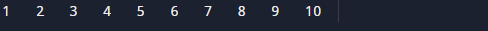Natural numbers As such, it is a whole, non-negative are all number 1, 2, 3, 4,5,6,7,8,9,10… They are usually counting  number and they will continue to infinity.while Whole number are all natural numbers including 0.

## C++ program to Find Sum of Natural Numbers using Recursion.

Firstly we declare required header file and variable and also initiates required values in variable. Next we take value from user at run time and then after we will find the sum of all natural number to given value using Recursion..

## Output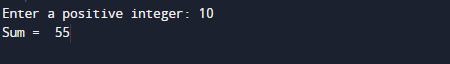In the above program, we have first declared and initialized a set variables required in the program.

• num=to take value from user.

In this program first we take a value from user.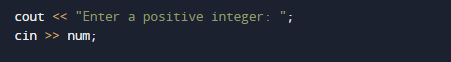After that in this Program we will pass the given number to function where we add all number using Recursion (adding all natural number from 1 to 10).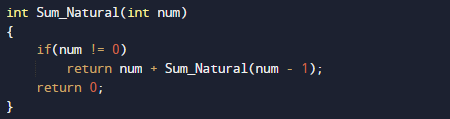Within the Function, we add all values and return num + Sum_Natural(num – 1).
After calculating values using recursion function returns sum or natural number back to main function where we print sum of all natural number.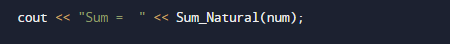In this tutorial we have learn about the C++ program to Find Sum of Natural Numbers using Recursion and its application with practical example. I hope you will like this tutorial.